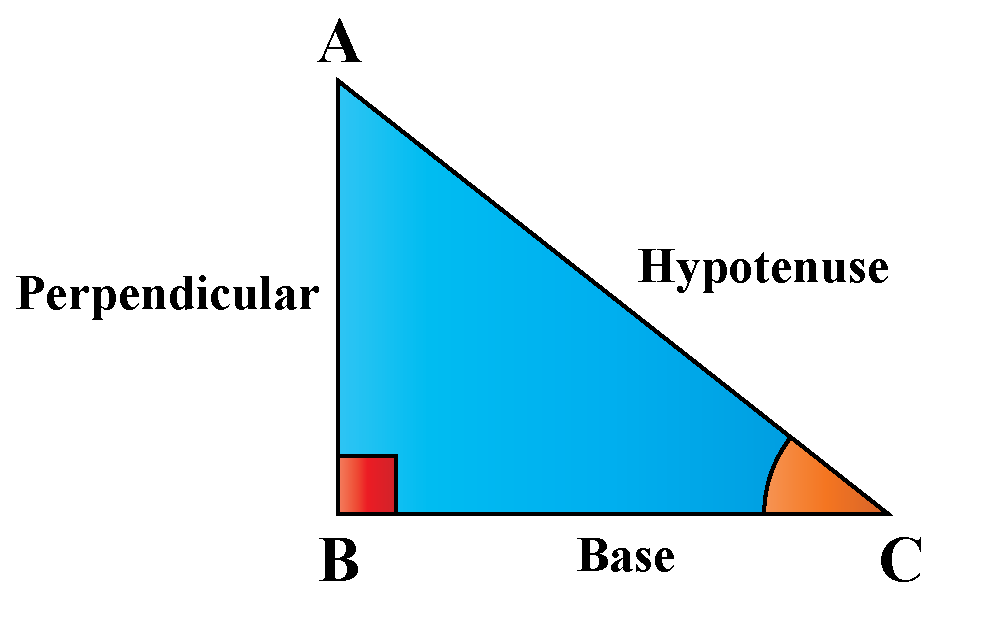# Trigonometry Formula

Trigonometry Formula
Go back to  'Trigonometry'

Trigonometry is the algebra of ratios of the sides of a triangle. The word Trigonometry was derived from greek, with trignos meaning triangle and metry meaning to measure. The earlier study of trigonometry started in the 2nd millennium BC by an Egyptian mathematician by the name Papyrus. Trigonometry finds its applications in other areas of mathematics such as calculus, coordinate geometry, plane geometry, and algebra.In this mini-lesson, we shall explore the world of trigonometry, by finding answers to questions like what are trigonometric functions, what are complementary angles, what are inverse trigonometric functions, and by understanding the use of these trigonometric ratios by solving examples and interactive questions.

## Lesson Plan

 1 What Are the Trigonometric Functions? 2 Important Notes on Trigonometric Functions 3 Think Out of the Box! 4 Solved Examples on Trigonometric Functions 5 Interactive Questions on Trigonometric Functions

## Trigonometric Functions

Trigonometry is the algebra of ratios of sides of a right-angled traingle. These ratios are represented as trigonometric ratios of sine(sin), cosine(cos), tangent(tan), cotangent(cot), secant(sec), cosecant(cosec).The Pythagoras theorem is helpful to build the relationship between the three sides of a right-angled triangle.

$\text {Hypotenuse}^2 = \text{Perpendicular}^2 + \text{Base}^2$

Further, the ratio of the sides of a right-angled triangle gives the following trigonometric ratios.

Trigonometric Ratios Ratio of Sides
$$\text{Sin }\theta$$ $$\dfrac{\text{Perpendicular}}{\text {Hypotenuse}}$$
$$\text{Cosec }\theta$$ $$\dfrac{\text {Hypotenuse}}{\text{Perpendicular}}$$
$$\text{Cos }\theta$$ $$\dfrac{\text{Base}}{\text {Hypotenuse}}$$
$$\text{Sec }\theta$$ $$\dfrac{\text {Hypotenuse}}{\text{Base}}$$
$$\text{Tan }\theta$$ $$\dfrac{\text{Perpendicular}}{\text{Base}}$$
$$\text{Cot }\theta$$ $$\dfrac{\text{Base}}{\text{Perpendicular}}$$

## Complementary angles

The two angles which sum up to a $$90^\circ$$ are called complementary angles.

Here the angle $$\theta$$ is an acute angle.

The trigonometry ratio formulae for complementary angles is as follows.

• $$\text{Sin }(90^\circ - \theta) = \text{Cos }\theta$$  also $$\text{Cos }(90^\circ - \theta) = \text{Sin }\theta$$
• $$\text{Tan }(90^\circ - \theta) = \text{Cot }\theta$$  also $$\text{Cot }(90^\circ - \theta) = \text{Tan }\theta$$
• $$\text{Cosec }(90^\circ -\theta) = \text{Sec }\theta$$  also $$\text{Sec }(90^\circ - \theta) = \text{Cosec }\theta$$

More Important Topics
Numbers
Algebra
Geometry
Measurement
Money
Data
Trigonometry
Calculus